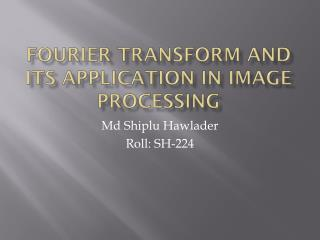DownloadDownload PresentationFourier Transform and its Application in Image Processing

# Fourier Transform and its Application in Image Processing

Download Presentation## Fourier Transform and its Application in Image Processing

- - - - - - - - - - - - - - - - - - - - - - - - - - - E N D - - - - - - - - - - - - - - - - - - - - - - - - - - -
##### Presentation Transcript

1. Fourier Transform and its Application in Image Processing Md Shiplu Hawlader Roll: SH-224

2. Overview • Fourier Series Theorem • Fourier Transform • Discrete Fourier Transform • Fast Fourier Transform

3. Fourier Series Theorem Any periodic function can be expressed as a weighted sum (infinite) of sine and cosine functions of varying frequency

4. Fourier Series

5. Fourier Transform • Transforms a signal (i.e., function) from the spatialdomain to the frequency domain. where

6. Discrete Fourier Transform (DFT)

7. Discrete Fourier Transform (DFT) • Forward DFT • Inverse DFT

8. Visualizing DFT • Typically, we visualize |F(u,v)| • The dynamic range of |F(u,v)| is typically very large • Apply stretching: (c is const) original image before scaling after scaling

9. Magnitude and Phase of DFT (1/2) magnitude phase

10. Magnitude and Phase of DFT (2/2) Reconstructed image using magnitude only (i.e., magnitude determines the contribution of each component!) Reconstructed image using phase only (i.e., phase determines which components are present!)

11. Why is FT Useful? • Easier to remove undesirable frequencies. • Faster perform certain operations in the frequencydomain than in the spatialdomain.

12. Removing undesirable frequencies frAequencies noisy signal To remove certain frequencies, set their corresponding F(u) coefficients to zero! remove high frequencies reconstructed signal

13. How do frequencies show up in an image? • Low frequencies correspond to slowly varying information (e.g., continuous surface). • High frequencies correspond to quickly varying information (e.g., edges) Original Image Low-passed

14. Example of noise reduction using FT

15. Frequency Filtering Steps 1. Take the FT of f(x): 2. Remove undesired frequencies: 3. Convert back to a signal:

16. Fast Fourier Transform (FFT) • The FFT is an efficient algorithm for computing the DFT • The FFT is based on the divide-and-conquer paradigm: • If n is even, we can divide a polynomial into two polynomials and we can write

17. The FFT Algorithm The running time is O(n log n)

18. Conclusion Fourier Transform has multitude of applications in all the field of engineering but has a tremendous contribution in image processing fields like image enhancement and restoration.

19. References • Image Processing, Analysis and Machine Vision, chapter 6.2.3. Chapman and Hall, 1993 • The Image Processing Handbook, chapter 4. CRC Press, 1992 • Fundamentals of Electronic Image Processing, chapter 8.4. IEEE Press, 1996 • http://en.wikipedia.org/wiki/Fourier_transform

20. Thank You